c/c++的const说明符——深入挖掘

const变量的文件作用域

• 在不同的.cpp文件内可以定义相同名称的const 对象。
• 如果要使const 我修饰的变量具有全局使用域，在定义该变量时，需要加上extern 关键字。

不使用extern关键字定义const变量

const int a = 100;

extern const int a;
int main() {
cout << a << endl;
return 0;
}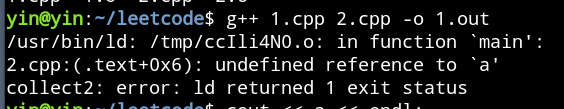使用extern关键字定义const 变量

extern const int a = 100;

extern const int a;
int main() {
cout << a << endl;
return 0;
}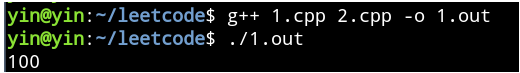进一步分析原因

• 第一步：新建一个1.cpp文件，定义如下：

const int value1 = 100;
extern const int value2 = 100;
int value3 = 100;
extern int value4 = 100;

• 第二步， 编译成.o文件。（报了一个编译警告，不管它）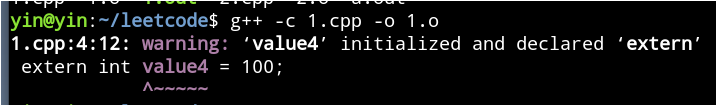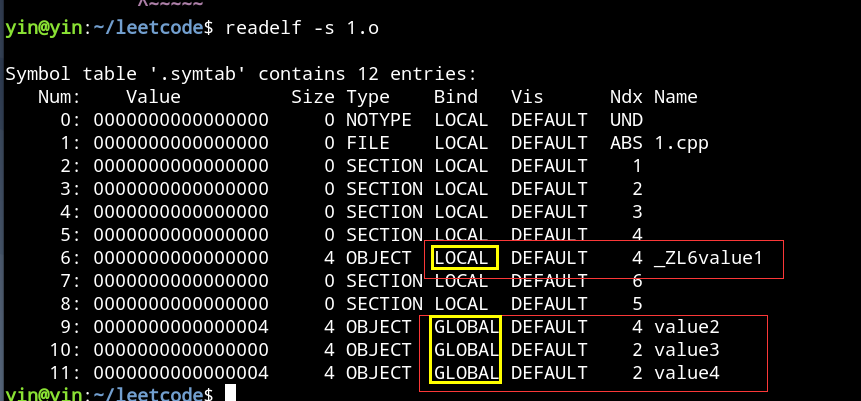修改const修饰的变量会发生什么

修改const修饰的全局变量

1 #include <iostream>
2 using namespace std;
3
4 const double a = 10.5;
5
6 int main() {
7     double* p = const_cast<double*>(&a);
8     *p = 110.5;
9     return 0;
10 }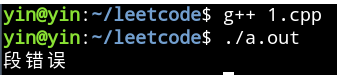1 #include <iostream>
2 using namespace std;
3
4 const int a = 10.5;
5 const int b = 10.5;
6
7 int c = 100;
8 int d = 100;
9
10 int main() {
11     cout << &a << endl;
12     cout << &b << endl;
13     cout << &c << endl;
14     cout << &d << endl;
15     return 0;
16 }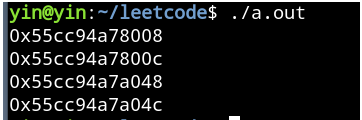修改const修饰的局部变量

1 #include <iostream>
2 using namespace std;
3
4 int main() {
5     const double a = 10.5;
6     double* p = const_cast<double*>(&a);
7     *p = 20.5;
8     cout << a << endl;
9     cout << *p << endl;
10     return 0;
11 }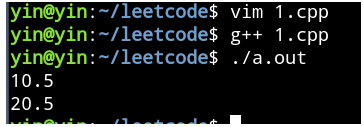1 #include <iostream>
2 using namespace std;
3
4 int main() {
5     volatile const double a = 10.5;
6     double* p = const_cast<double*>(&a);
7     *p = 20.5;
8     cout << a << endl;
9     cout << *p << endl;
10     return 0;
11 }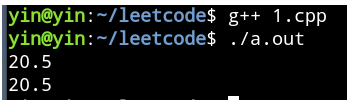c++的const属性为bitwise

什么是bitwise-const

1 #include <iostream>
2
3 using namespace std;
4
5 struct Info{
6     int& a;
7     int* p;
8 };
9
10 void Modify(const Info& input) {
11     input.a += 100;
12     *input.p += 100;
13 }
14
15 int main() {
16     int num1 = 100;
17     int num2 = 100;
18     Info input{num1, &num2};
19     cout << input.a << endl;
20     cout << *input.p << endl;
21
22     Modify(input);
23
24     cout << input.a << endl;
25     cout << *input.p << endl;
26     return 0;
27 }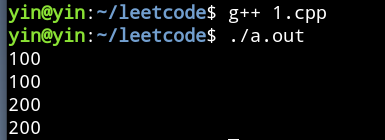如何取某一个成员变量的const属性

1 #include <iostream>
2
3 using namespace std;
4
5 struct Info{
6     int a;
7     int b;
8 };
9
10 int main() {
11     const Info data{10, 20};
12     data.a = 100;
13     data.b = 100;
14     cout << data.a << " " << data.b << endl;
15     return 0;
16 }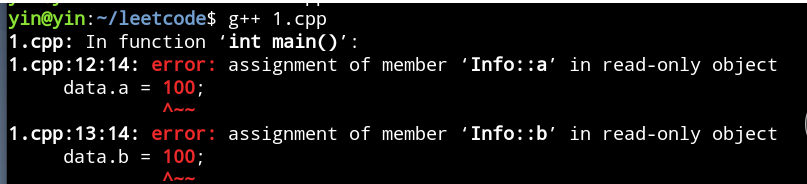1 #include <iostream>
2
3 using namespace std;
4
5 struct Info{
6     mutable int a;
7     mutable int b;
8 };
9
10 int main() {
11     const Info data{10, 20};
12     data.a = 100;
13     data.b = 100;
14     cout << data.a << " " << data.b << endl;
15     return 0;
16 }posted @ 2021-05-04 17:00  殷大侠  阅读(127)  评论(0编辑  收藏  举报